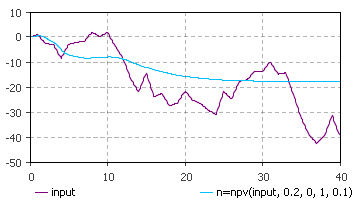AnyLogic

# npv

npv(stream, discountRate, initialValue, factor, timeStep) is the system dynamics function that returns the net present value of stream computed using discountRate. The initial value is determined by initialValue (usually 0) and the value is reported after multiplying by factor (usually 1).

The plot on the figure below illustrates how the delay function works:This function can be called in formulas of system dynamics variables.

#### Units

stream — unit

discountRate — 1/time

initialValue — unit*time

factor — dimensionless

timeStep — time

npv() — unit*time